## 搶先體驗長城風駿7 高端路線皮卡舒適氣派

2018年11月01日 11:38 來源：車行天下 超過：8532次關注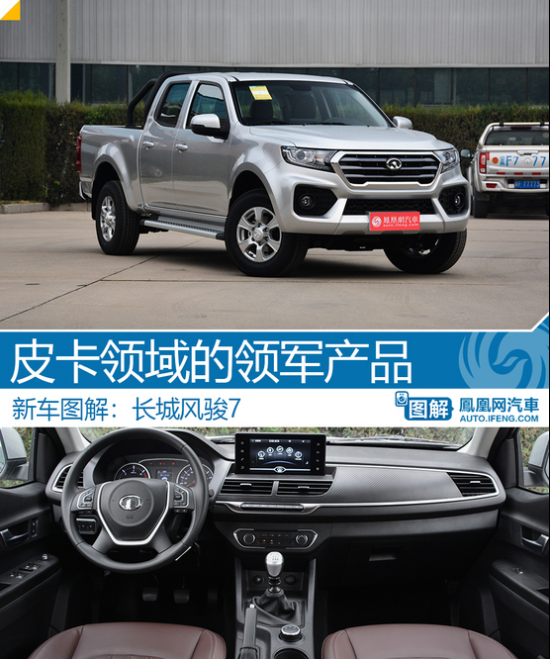長城集團在SUV領域，其驚人的銷量，讓哈弗品牌成為了SUV的領導者。但很多人并不知道，長城品牌的誕生最初也是由皮卡起家，從上世紀末推出的第一款車叫做長城迪爾。20多年過去了，由于受到國家政策限制，皮卡一直沒有較大的發展。而長城在這個偏向小眾的市場上，仍然顯示出了一枝獨秀。多年來，長城旗下的風駿系列在國內外的市場上都獲得了不小的成功。而這次，長城推出的風駿7系列，就是要讓皮卡車型顯得更為高端。其豐富的配置，接近于主流的轎車與SUV，讓這樣一款工具車的駕乘體驗更為舒適。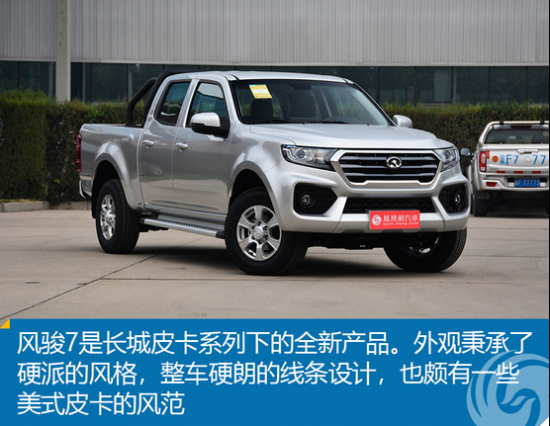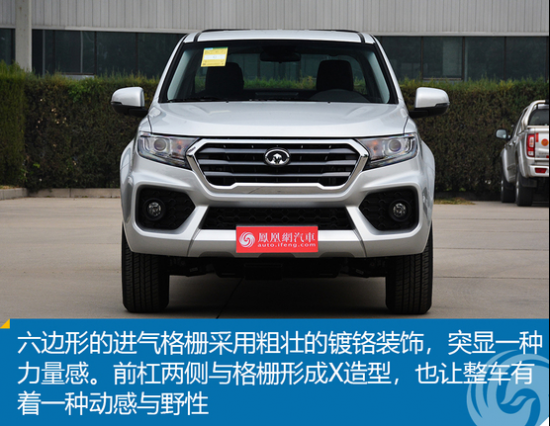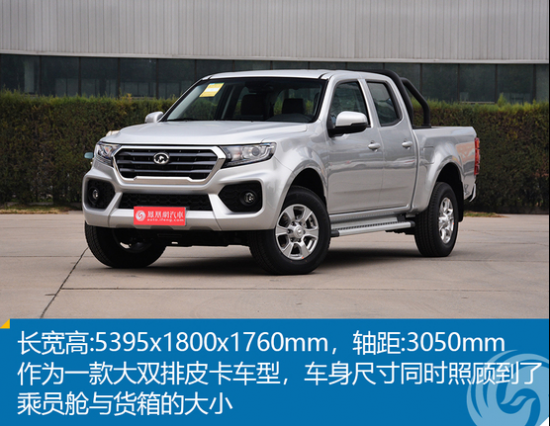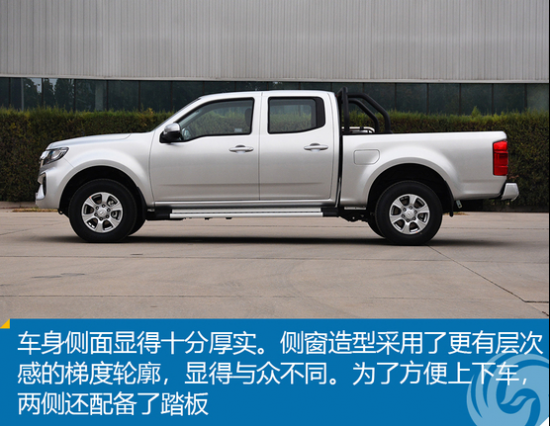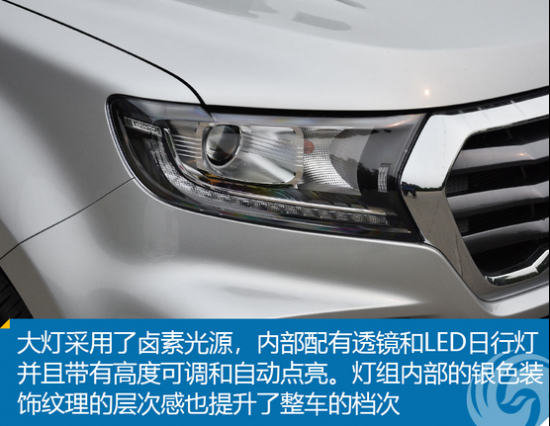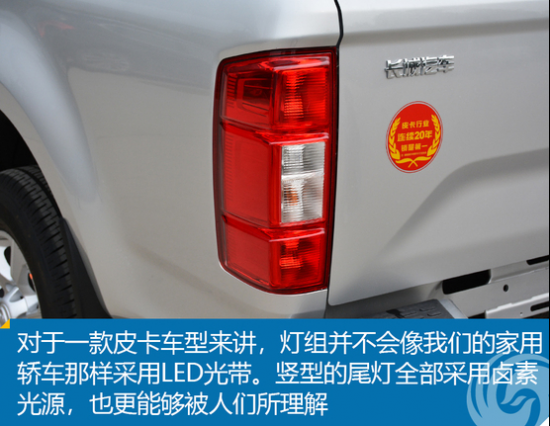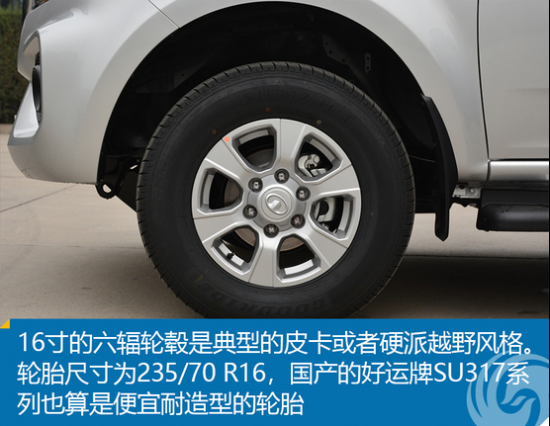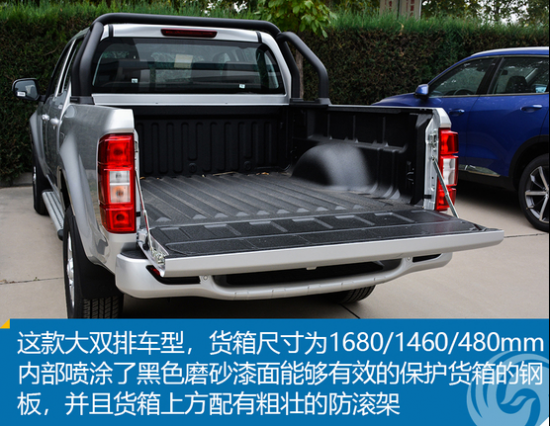相比其風駿之前的車型，風駿7看起來更為霸氣十足。車頭姿態很高，整車采用更加硬朗的線條，硬派的設計元素更顯美式皮卡的風范。大燈與格柵的設計也更加時尚，貼近于主流。作為一款大雙排皮卡，整車車身長度將近5米4，在駕乘空間與拉貨能力方面，都有著突出的表現。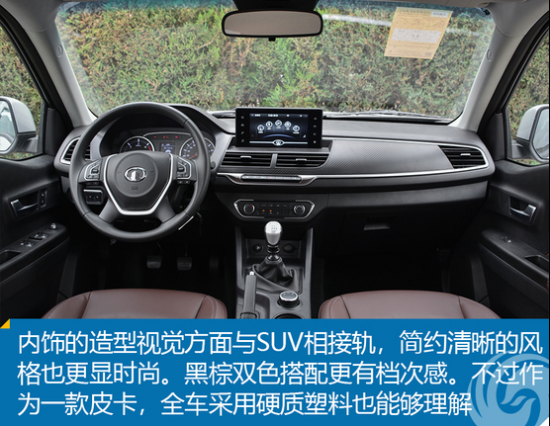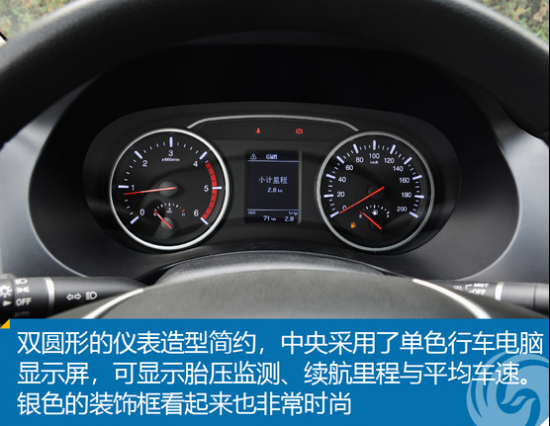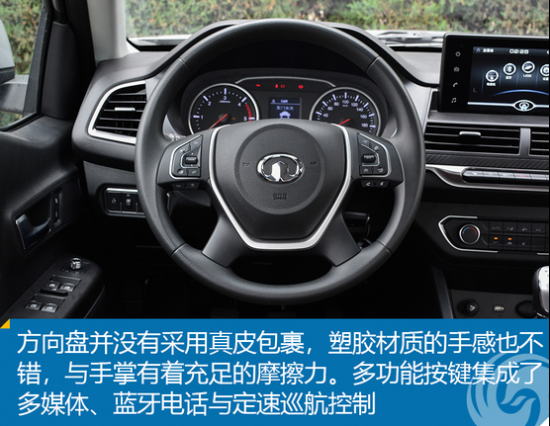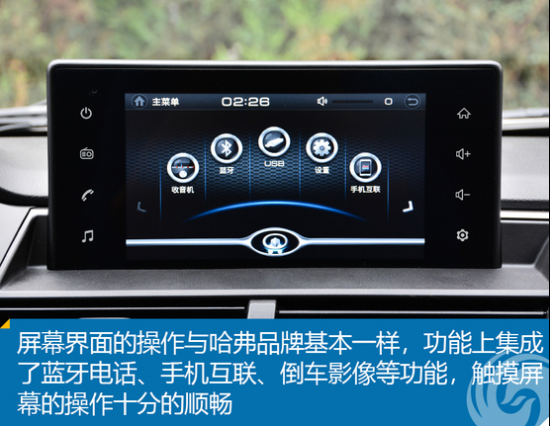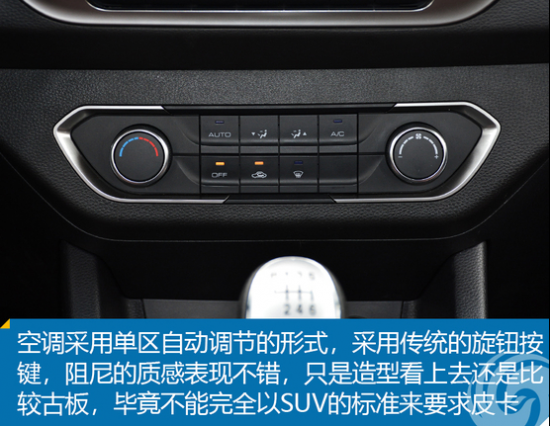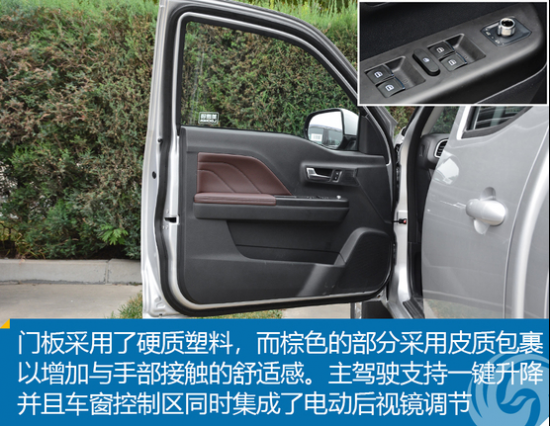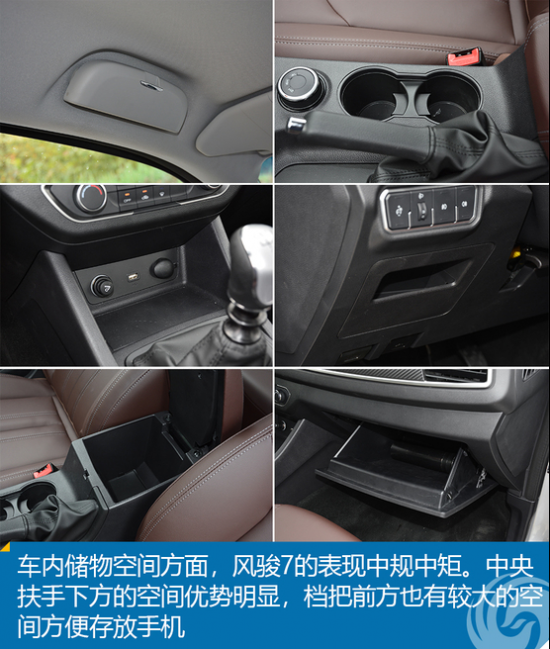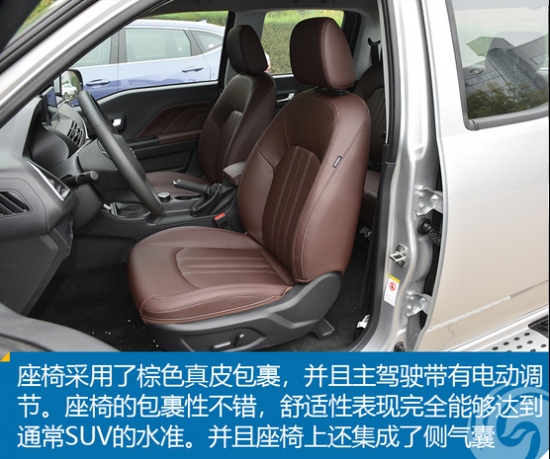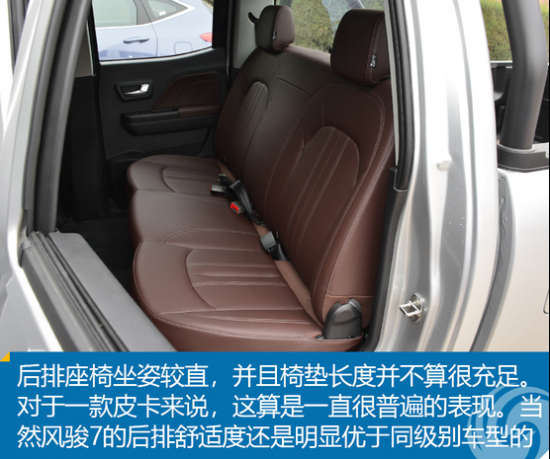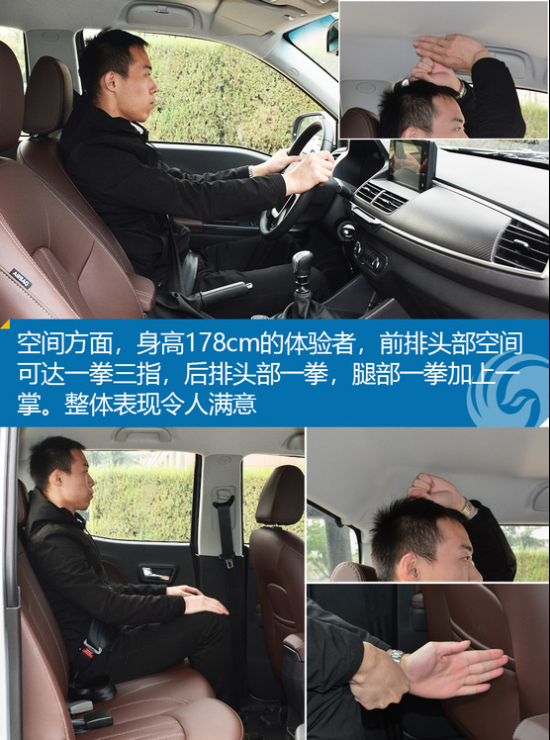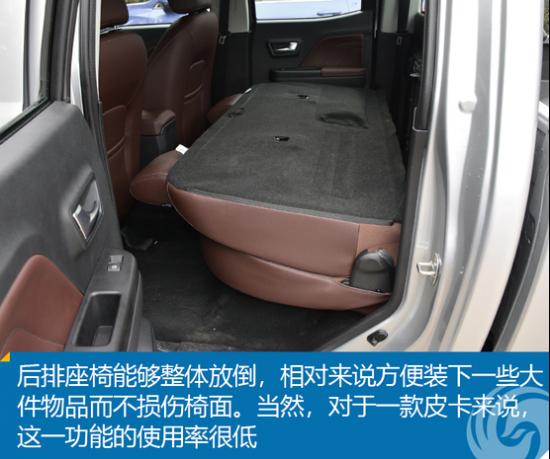內飾方面，雖然風駿7并沒有采用軟質搪塑，這對于皮卡來說也并無必要。而內飾的整體設計更偏向如今的SUV車型，設計簡潔而有層次，配色與視覺質感更顯檔次。中央大屏與哈弗品牌采用了相近的UI設計，操作起來十分順手。而對于這樣一款車身很長的皮卡來說，倒車影像這樣的配置也非常的必要。當然，全車帶有側氣囊和氣簾，并標配ESP，對安全負責任的態度，是很多同級別國產皮卡并不能夠做到的。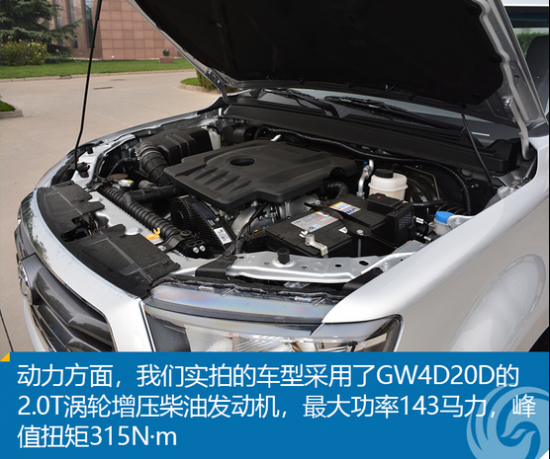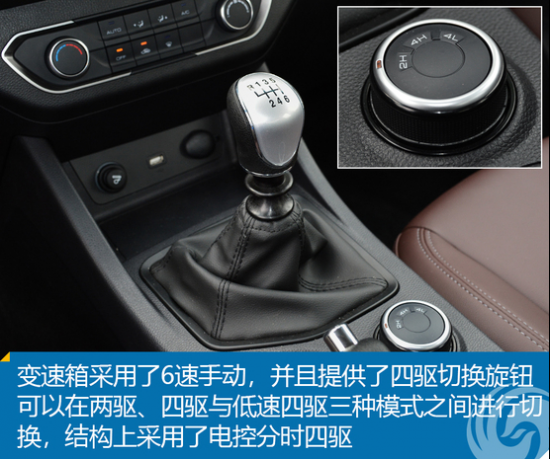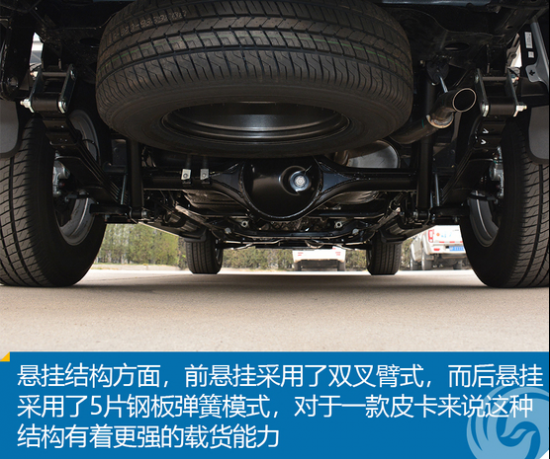總結：雖說在多數人的眼里，皮卡只是一款工具車，并沒有必要做得這么豪華舒適。不過時代在發展，中國的鄉村的經濟水平早已極大的改善，人們對于新車的舒適性、配置方面要求越來越高。當然，作為一款皮卡車型，也是很多越野玩家的最愛，出色的四驅能力也是風駿7的一大優勢。而將很多轎車或SUV上才會有的配置，例如中控大屏、手機互聯、自動大燈、倒車影像、座椅加熱等功能加持在這樣一臺皮卡上，其出色的產品力，能夠再次創造中國皮卡領域的新標桿。

#### 相關文章

0-500 字已有評論 0條 查看評論>>

### 熱門標簽

?
• 快速找車
• 選擇品牌
• 選擇品牌
• A  奧迪
• A  阿斯頓·馬丁
• A  阿爾法·羅密歐
• B  寶沃
• B  布加迪
• B  巴博斯
• B  保時捷
• B  賓利
• B  奔馳
• B  寶馬
• B  本田
• B  別克
• B  標致
• B  比亞迪
• B  寶駿
• B  北汽制造
• B  北汽新能源
• B  北汽幻速
• B  北汽威旺
• B  北京汽車
• B  奔騰
• B  北汽紳寶
• C  長安
• C  長安商用
• C  長城
• C  昌河
• D  大眾
• D  道奇
• D  DS
• D  東南
• D  東風風神
• D  東風風行
• D  東風小康
• D  東風風度
• D  東風
• F  福特
• F  豐田
• F  菲亞特
• F  法拉利
• F  福田
• F  福迪
• F  福汽啟騰
• G  觀致
• G  廣汽傳祺
• G  廣汽吉奧
• G  GMC
• H  紅旗
• H  漢騰汽車
• H  哈弗
• H  哈飛
• H  海格
• H  海馬
• H  華頌
• H  黃海
• H  華泰
• H  恒天
• J  吉利汽車
• J  捷豹
• J  Jeep
• J  江淮
• J  江鈴
• J  金杯
• J  九龍
• J  金旅
• K  凱翼
• K  凱迪拉克
• K  克萊斯勒
• K  科尼塞克
• K  卡威
• K  開瑞
• L  路虎
• L  林肯
• L  勞斯萊斯
• L  蘭博基尼
• L  雷克薩斯
• L  鈴木
• L  雷諾
• L  理念
• L  力帆
• L  蓮花汽車
• L  獵豹
• L  路特斯
• L  陸風
• M  馬自達
• M  MG
• M  MINI
• M  瑪莎拉蒂
• M  摩根
• M  邁凱輪
• N  納智捷
• O  歐寶
• O  謳歌
• O  歐朗
• Q  奇瑞
• Q  起亞
• Q  啟辰
• R  日產
• R  榮威
• R  瑞麒
• S  三菱
• S  斯威汽車
• S  薩博
• S  smart
• S  斯柯達
• S  斯巴魯
• S  思銘
• S  雙龍
• S  上汽大通
• S  雙環
• T  特斯拉
• T  騰勢
• W  沃爾沃
• W  五菱汽車
• W  五十鈴
• W  威茲曼
• W  威麟
• X  現代
• X  雪佛蘭
• X  雪鐵龍
• X  西雅特
• Y  一汽
• Y  英菲尼迪
• Y  英致
• Y  依維柯
• Y  野馬汽車
• Y  永源
• Z  眾泰
• Z  中華
• Z  中興
• Z  知豆
• 選擇車系
• 選擇車系
• 車型對比
• 選擇品牌
• 選擇品牌
• A  奧迪
• A  阿斯頓·馬丁
• A  阿爾法·羅密歐
• B  寶沃
• B  布加迪
• B  巴博斯
• B  保時捷
• B  賓利
• B  奔馳
• B  寶馬
• B  本田
• B  別克
• B  標致
• B  比亞迪
• B  寶駿
• B  北汽制造
• B  北汽新能源
• B  北汽幻速
• B  北汽威旺
• B  北京汽車
• B  奔騰
• B  北汽紳寶
• C  長安
• C  長安商用
• C  長城
• C  昌河
• D  大眾
• D  道奇
• D  DS
• D  東南
• D  東風風神
• D  東風風行
• D  東風小康
• D  東風風度
• D  東風
• F  福特
• F  豐田
• F  菲亞特
• F  法拉利
• F  福田
• F  福迪
• F  福汽啟騰
• G  觀致
• G  廣汽傳祺
• G  廣汽吉奧
• G  GMC
• H  紅旗
• H  漢騰汽車
• H  哈弗
• H  哈飛
• H  海格
• H  海馬
• H  華頌
• H  黃海
• H  華泰
• H  恒天
• J  吉利汽車
• J  捷豹
• J  Jeep
• J  江淮
• J  江鈴
• J  金杯
• J  九龍
• J  金旅
• K  凱翼
• K  凱迪拉克
• K  克萊斯勒
• K  科尼塞克
• K  卡威
• K  開瑞
• L  路虎
• L  林肯
• L  勞斯萊斯
• L  蘭博基尼
• L  雷克薩斯
• L  鈴木
• L  雷諾
• L  理念
• L  力帆
• L  蓮花汽車
• L  獵豹
• L  路特斯
• L  陸風
• M  馬自達
• M  MG
• M  MINI
• M  瑪莎拉蒂
• M  摩根
• M  邁凱輪
• N  納智捷
• O  歐寶
• O  謳歌
• O  歐朗
• Q  奇瑞
• Q  起亞
• Q  啟辰
• R  日產
• R  榮威
• R  瑞麒
• S  三菱
• S  斯威汽車
• S  薩博
• S  smart
• S  斯柯達
• S  斯巴魯
• S  思銘
• S  雙龍
• S  上汽大通
• S  雙環
• T  特斯拉
• T  騰勢
• W  沃爾沃
• W  五菱汽車
• W  五十鈴
• W  威茲曼
• W  威麟
• X  現代
• X  雪佛蘭
• X  雪鐵龍
• X  西雅特
• Y  一汽
• Y  英菲尼迪
• Y  英致
• Y  依維柯
• Y  野馬汽車
• Y  永源
• Z  眾泰
• Z  中華
• Z  中興
• Z  知豆
• 選擇車系
• 選擇車系
• 選擇車型
• 選擇車型
• 意見反饋